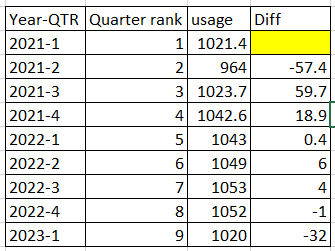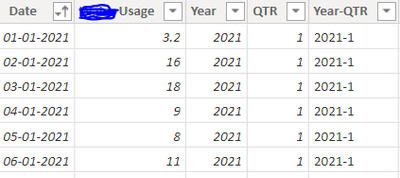cancel
Showing results for
Did you mean:

Fabric is Generally Available. Browse Fabric Presentations. Work towards your Fabric certification with the Cloud Skills Challenge.Helper I

## How to calculate difference between current quarter and previous quarter

Hi, can somebody help me with this.

Available data

 Year-QTR Quarter rank usage 2021-1 1 1021.4 2021-2 2 964 2021-3 3 1023.7 2021-4 4 1042.6 2022-1 5 1043 2022-2 6 1049 2022-3 7 1053 2022-4 8 1052 2023-1 9 1020

Expected resultThe differnce for the first (Base) value must be blank.

Any help would be much appriciated. Thanks in advance.

3 ACCEPTED SOLUTIONSSuper User

@Reddy5833 Try:

``````Measure =
VAR __Current = SUM('Table'[usage])
VAR __CurrentQR = MAX('Table'[Quarter rank])
VAR __Previous = SUMX(FILTER(ALL('Table'),[Quarter rank] = __CurrentQR - 1),[usage])
VAR __Result = IF(__Previous = BLANK(),BLANK(),__Current - __Previous)
RETURN
__Result``````

Become an expert!: Enterprise DNA
External Tools: MSHGQM
Latest book!:
Mastering Power BI 2nd EditionDAX is easy, CALCULATE makes DAX hard...Helper I

@Greg_Deckler Thanks a lot, it works perfect..Helper I

I got the answer to my question, I found it from your video ( MSHGQM - Power BI DAX - Don't Use CALCULATE!

Measure to be used when there are multiple categories.

Measure 1 =
VAR __Site = VALUES('Sample'[Site Name])
VAR __Current = sum('Sample'[Usage])
VAR __Table = FILTER(ALLSELECTED('Sample'),'Sample'[Site Name] in  __Site)
VAR __CurrentQR = MAX('Sample'[YQRank])
VAR __Previous = SUMX(FILTER(__Table,[YQRank] = __CurrentQR - 1), [Usage])
VAR __Result = IF(__Current=BLANK(),"0",__Current - __Previous)
RETURN
__Result
7 REPLIES 7Helper I

Hi @Greg_Deckler, I have usage data for 5 years, (around 1500 records), the table contains date field, formateed as date data type only, I want to calculate differnce of usage between current qtr and prvs qtr (same as i asked in previos question, but based on proper date field)

I'm using below measeu to calculate prev quarter usage,

but it is not giving the result as i expected unless I connect with date table (though I'm not using any field from date table in the measure )

Prev QTR Usage = CALCULATE(SUM('Sample'[Usage]), DATEADD('Sample'[Date],-1,QUARTER))
This measure is giving blanks, if i dont connect with date table.

measure to calculate diff: -- works fine when i connect with date table

Diff =
)

Measure to get first date as blank -- it is not working even if i connect with date table, giving same result as above

Diff 1 =
IF(SUM('Sample'[YQ Rank])=1, BLANK(),
)

sample dataresult when connected with date dimensionResult when not connected with dateSuper User

@Reddy5833 This is because you are using CALCULATE. CALCULATE almost requires a star schema to work properly. CALCULATE has a really hard time dealing with single table data models so you get wacky results. See this video:

Become an expert!: Enterprise DNA
External Tools: MSHGQM
Latest book!:
Mastering Power BI 2nd EditionDAX is easy, CALCULATE makes DAX hard...Helper I

Hi @Greg_Deckler , I saw the video, it's brilliant.. Thanks a lotSuper User

@Reddy5833 Try:

``````Measure =
VAR __Current = SUM('Table'[usage])
VAR __CurrentQR = MAX('Table'[Quarter rank])
VAR __Previous = SUMX(FILTER(ALL('Table'),[Quarter rank] = __CurrentQR - 1),[usage])
VAR __Result = IF(__Previous = BLANK(),BLANK(),__Current - __Previous)
RETURN
__Result``````

Become an expert!: Enterprise DNA
External Tools: MSHGQM
Latest book!:
Mastering Power BI 2nd EditionDAX is easy, CALCULATE makes DAX hard...Helper I

Hi @Greg

Hope you are doing good

The Measure you share is working fine when I have only one category, but when I have multipel categories in my dataset it is unable to find the difference between current quarter and previous quarter when I choose a category from the slicer.

But it works fine when we select all categories from slicer it is showing the over all differnce between current quarter and prev quarter.

I'm unable to use calculate function in the measure it is giving wrong values if I do so

Below is the measre you shared a while ago.

```Measure =
VAR __Current = SUM('Table'[usage])
VAR __CurrentQR = MAX('Table'[Quarter rank])
VAR __Previous = SUMX(FILTER(ALL('Table'),[Quarter rank] = __CurrentQR - 1),[usage])
VAR __Result = IF(__Previous = BLANK(),BLANK(),__Current - __Previous)
RETURN
__Result```Helper I

@Greg_Deckler Thanks a lot, it works perfect..Helper I

I got the answer to my question, I found it from your video ( MSHGQM - Power BI DAX - Don't Use CALCULATE!

Measure to be used when there are multiple categories.

Measure 1 =
VAR __Site = VALUES('Sample'[Site Name])
VAR __Current = sum('Sample'[Usage])
VAR __Table = FILTER(ALLSELECTED('Sample'),'Sample'[Site Name] in  __Site)
VAR __CurrentQR = MAX('Sample'[YQRank])
VAR __Previous = SUMX(FILTER(__Table,[YQRank] = __CurrentQR - 1), [Usage])
VAR __Result = IF(__Current=BLANK(),"0",__Current - __Previous)
RETURN
__ResultAnnouncements#### Power BI Monthly Update - November 2023

Check out the November 2023 Power BI update to learn about new features.#### Fabric Community News unified experience

Read the latest Fabric Community announcements, including updates on Power BI, Synapse, Data Factory and Data Activator.#### The largest Power BI and Fabric virtual conference

130+ sessions, 130+ speakers, Product managers, MVPs, and experts. All about Power BI and Fabric. Attend online or watch the recordings.Top Solution Authors
Top Kudoed Authors
Users online (3,314)